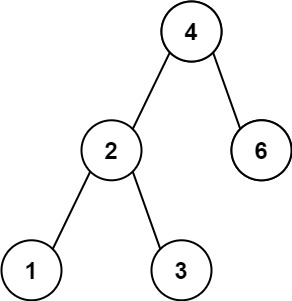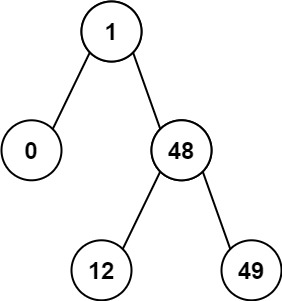## 783. Minimum Distance Between BST Nodes

Given the `root` of a Binary Search Tree (BST), return the minimum difference between the values of any two different nodes in the tree.

Note: This question is the same as 530: https://leetcode.com/problems/minimum-absolute-difference-in-bst/

Example 1:```Input: root = [4,2,6,1,3]
Output: 1
```

Example 2:```Input: root = [1,0,48,null,null,12,49]
Output: 1
```

Constraints:

• The number of nodes in the tree is in the range `[2, 100]`.
• `0 <= Node.val <= 105`

## Rust Solution

``````struct Solution;
use rustgym_util::*;

trait Inorder {
fn inorder(&self, prev: &mut Option<i32>, min: &mut i32);
}

fn inorder(&self, prev: &mut Option<i32>, min: &mut i32) {
if let Some(node) = self {
let node = node.borrow();
Self::inorder(&node.left, prev, min);
if let Some(prev_val) = prev.as_mut() {
*min = i32::min((node.val - *prev_val).abs(), *min);
*prev_val = node.val;
} else {
*prev = Some(node.val);
}
Self::inorder(&node.right, prev, min);
}
}
}

impl Solution {
fn min_diff_in_bst(root: TreeLink) -> i32 {
let mut min = std::i32::MAX;
let mut prev: Option<i32> = None;
root.inorder(&mut prev, &mut min);
min
}
}

#[test]
fn test() {
let root = tree!(4, tree!(2, tree!(1), tree!(3)), tree!(6));
assert_eq!(Solution::min_diff_in_bst(root), 1);
}
``````

Having problems with this solution? Click here to submit an issue on github.